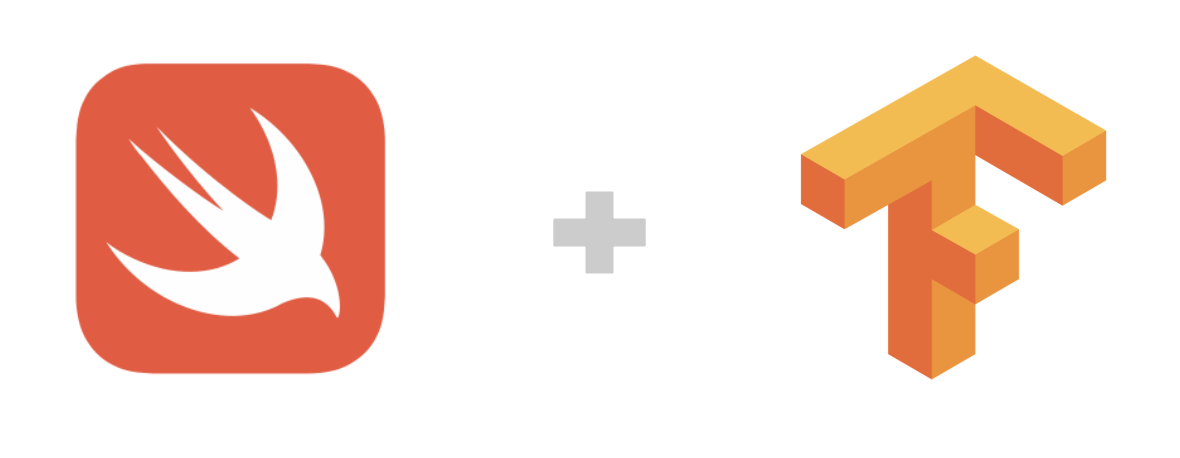# Swift for TensorFlow (S4TF)¶“Swift for Tensorflow is an attempt to change the default tools used by the entire machine learning and data science ecosystem.”

– Jameson Toole, Co-founder & CTO of Fritz.ai

## Introduction to S4TF¶

Google 推出的 Swift for TensorFlow （简称S4TF）是专门针对 TensorFlow 优化过的 Swift 版本。（目前处在 Pre-Alpha 阶段）

Swift 语言是 Chris Lattner 在苹果公司工作时创建的。 现在 Chris Lattner 在 Google Brain 工作，专门从事深度学习的研究，并为 Swift 重写了编译器，为 Tensorflow 做定制优化，

### Why using Swift for TensorFlow Development¶

1. 开发效率高：强类型语言，能够静态检查变量类型

2. 迁移成本低：与 Python，C，C++ 能够无缝结合

3. 执行性能高：能够直接编译为底层硬件代码

4. 专门为机器学习打造：语言原生支持自动微分系统

## S4TF environment configuration¶

Local installation of Swift for Tensorflow ——————————————’

### Express experience of Swift for TensorFlow in Colaboratory¶

Google 的 Colaboratory 可以直接支持 Swift 语言的运行环境。可以通过下面的链接，直接打开一个 Swift 运行环境的 Colab Notebook ，这是一个最方便立即可以体验 Swift for Tensorflow 的方法。

### Express experience of Swift for TensorFlow in Docker¶

• 获得一个已经安装好 S4TF 的 Swift REPL 环境

在命令行中执行 `docker run -it --privileged --userns=host zixia/swift swift`

• 获得一个 S4TF 的 Bash 终端

在命令行中执行 `docker run -it --privileged --userns=host zixia/swift bash` 来打开一个 Bash 终端

• 获得一个 S4TS 的 Jupyter Notebook

在命令行中执行 `nvidia-docker run -ti --rm -p 8888:8888 --cap-add SYS_PTRACE -v "\$(pwd)":/notebooks zixia/swift` 来启动 Jupyter ，然后根据提示的 URL ，打开浏览器访问即可。

## Basic usage of S4TF¶

Swift 是动态强类型语言，也就是说 Swift 支持通过编译器自动检测类型，同时要求变量的使用要严格符合定义，所有变量都必须先定义后使用。

```var n = 42
n = "string"
```

```Cannot assign value of type 'String' to type 'Int'
```

```import TensorFlow

// 声明两个Tensor
let x = Tensor<Float>()
let y = Tensor<Float>()

// 对两个 Tensor 做加法运算
let w = x + y

// 输出结果
print(w)
```

### Using standard TensorFlow API in Swift¶

```let x = Tensor<BFloat16>(zeros: [32, 128])
let h1 = sigmoid(x • w1 + b1)
let h2 = tanh(h1 • w1 + b1)
let h3 = softmax(h2 • w1 + b1)
```

```let imageBatch = Dataset(elements: images)
let labelBatch = Dataset(elements: labels)
let zipped = zip(imageBatch, labelBatch).batched(8)

let imageBatch = Dataset(elements: images)
let labelBatch = Dataset(elements: labels)
for (image, label) in zip(imageBatch, labelBatch) {
let y = image • w + b
let loss = (y - label).squared().mean()
print(loss)
}
```

Swift 语言支持直接加载 Python 函数库（比如 NumPy），也支持直接加载系统动态链接库，很方便的做到即导入即用。

```import Python

let np = Python.import("numpy")
let x = np.array([[1, 2], [3, 4]])
let y = np.array([11, 12])
print(x.dot(y))
```

```[35 81]
```

```import Glibc
let x = malloc(18)
memcpy(x, "memcpy from Glibc", 18)
free(x)
```

### Native support of automatic differentiation¶

```@differentiable
func frac(_ x:Double) -> Double {
return 1/x
}

gradient(at:0.5) { x in frac(x) }
```

```-4.0
```

### MNIST digit catagorization¶

```import TensorFlow
import Python
import Foundation

/**
* The Swift Module for MNIST Dataset:
* https://github.com/huan/swift-MNIST
*/
import MNIST

struct MLP: Layer {
typealias Input = Tensor<Float>
typealias Output = Tensor<Float>

var flatten = Flatten<Float>()
var dense = Dense<Float>(inputSize: 784, outputSize: 10)

@differentiable
public func callAsFunction(_ input: Input) -> Output {
return input.sequenced(through: flatten, dense)
}
}

var model = MLP()

let mnist = MNIST()
let ((trainImages, trainLabels), (testImages, testLabels)) = mnist.loadData()

let imageBatch = Dataset(elements: trainImages).batched(32)
let labelBatch = Dataset(elements: trainLabels).batched(32)

for (X, y) in zip(imageBatch, labelBatch) {
let logits = model(X)
return softmaxCrossEntropy(logits: logits, labels: y)
}

// Update parameters by optimizer
}

let logits = model(testImages)
let acc = mnist.getAccuracy(y: testLabels, logits: logits)

print("Test Accuracy: \(acc)" )
```

```Downloading train-images-idx3-ubyte ...